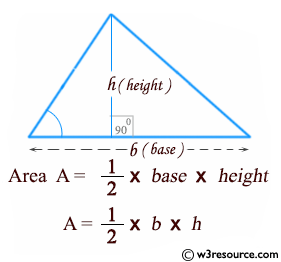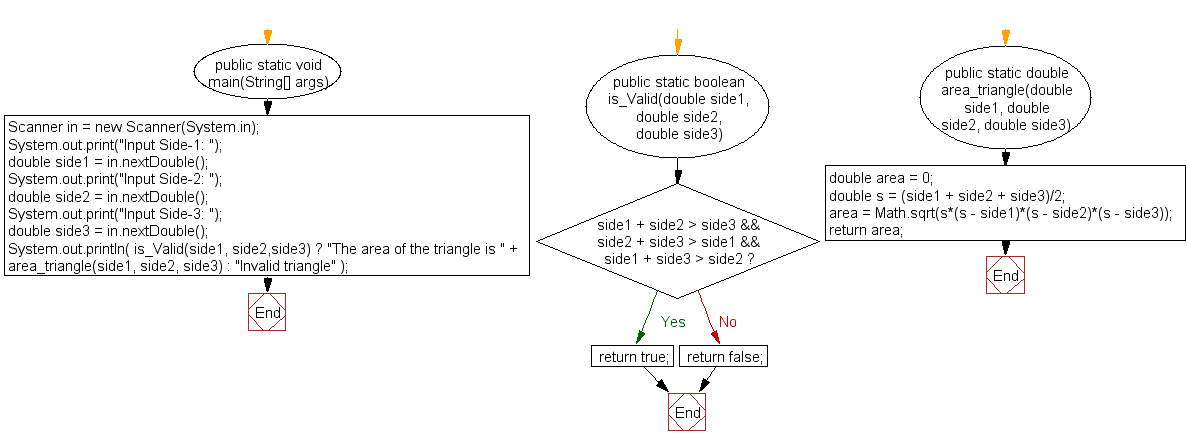﻿ Java exercises: Calculate the area of a triangle - w3resource# Java Method Exercises: Calculate the area of a triangle

## Java Method: Exercise-13 with Solution

Write Java methods to calculate the area of a triangle.

Pictorial Presentation:Sample Solution:

Java Code:

``````import java.util.Scanner;
public class Exercise13 {
public static void main(String[] args)
{
Scanner in = new Scanner(System.in);
System.out.print("Input Side-1: ");
double side1 = in.nextDouble();
System.out.print("Input Side-2: ");
double side2 = in.nextDouble();
System.out.print("Input Side-3: ");
double side3 = in.nextDouble();
System.out.println( is_Valid(side1, side2,side3) ?
"The area of the triangle is " + area_triangle(side1, side2, side3) : "Invalid triangle" );
}

public static boolean is_Valid(double side1, double side2, double side3) {
if( side1 + side2 > side3 &&
side2 + side3 > side1 &&
side1 + side3 > side2) return true;
else return false;
}
public static double area_triangle(double side1, double side2, double side3) {
double area = 0;
double s = (side1 + side2 + side3)/2;
area = Math.sqrt(s*(s - side1)*(s - side2)*(s - side3));
return area;
}
}
```
```

Sample Output:

```Input Side-1: 10
Input Side-2: 15
Input Side-3: 20
The area of the triangle is 72.61843774138907
```

Flowchart:Java Code Editor:

What is the difficulty level of this exercise?

﻿

## Java: Tips of the Day

Array vs ArrayLists:

The main difference between these two is that an Array is of fixed size so once you have created an Array you cannot change it but the ArrayList is not of fixed size. You can create instances of ArrayLists without specifying its size. So if you create such instances of an ArrayList without specifying its size Java will create an instance of an ArrayList of default size.

Once an ArrayList is full it re-sizes itself. In fact, an ArrayList is internally supported by an array. So when an ArrayList is resized it will slow down its performance a bit as the contents of the old Array must be copied to a new Array.

At the same time, it's compulsory to specify the size of an Array directly or indirectly while creating it. And also Arrays can store both primitives and objects while ArrayLists only can store objects.

Ref: https://bit.ly/3o8L2KH